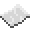# math.ldexp

Compute a floating point number from the given mantissa and exponent. This is the inverse operation of math.frexp, and computes $2{\text{mantissa}}^{\text{exponent}}$.ExampleTurn user input into a number
Read a two lines from the user, and compute the floating point number represented by the given mantissa and exponent.
Code
<nowiki>
print(math.ldexp(m, e))
</nowiki>

Output Depends on what the user wrote. For instance, if they were to enter 0.625 and 3, the number print would be 5

math.ldexp
Function
Syntax
math.ldexp(
• mantissa : number
• exponent : number
)

Returns number
API math
Source Lua (source)